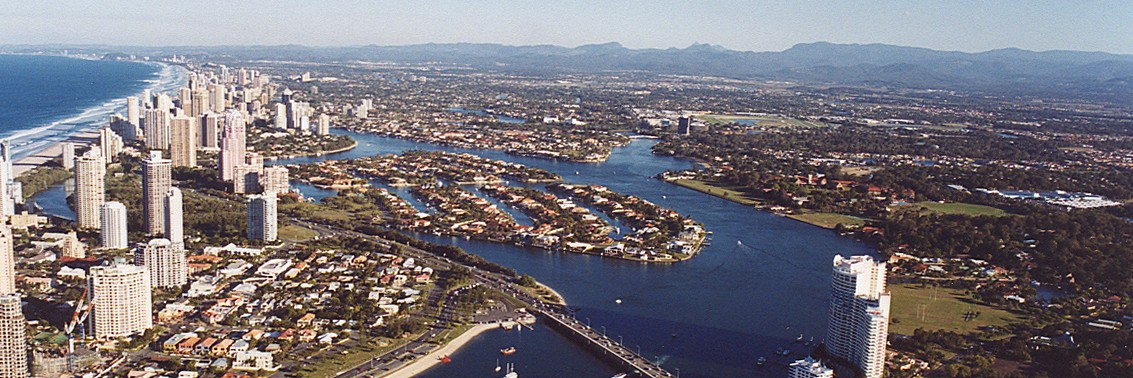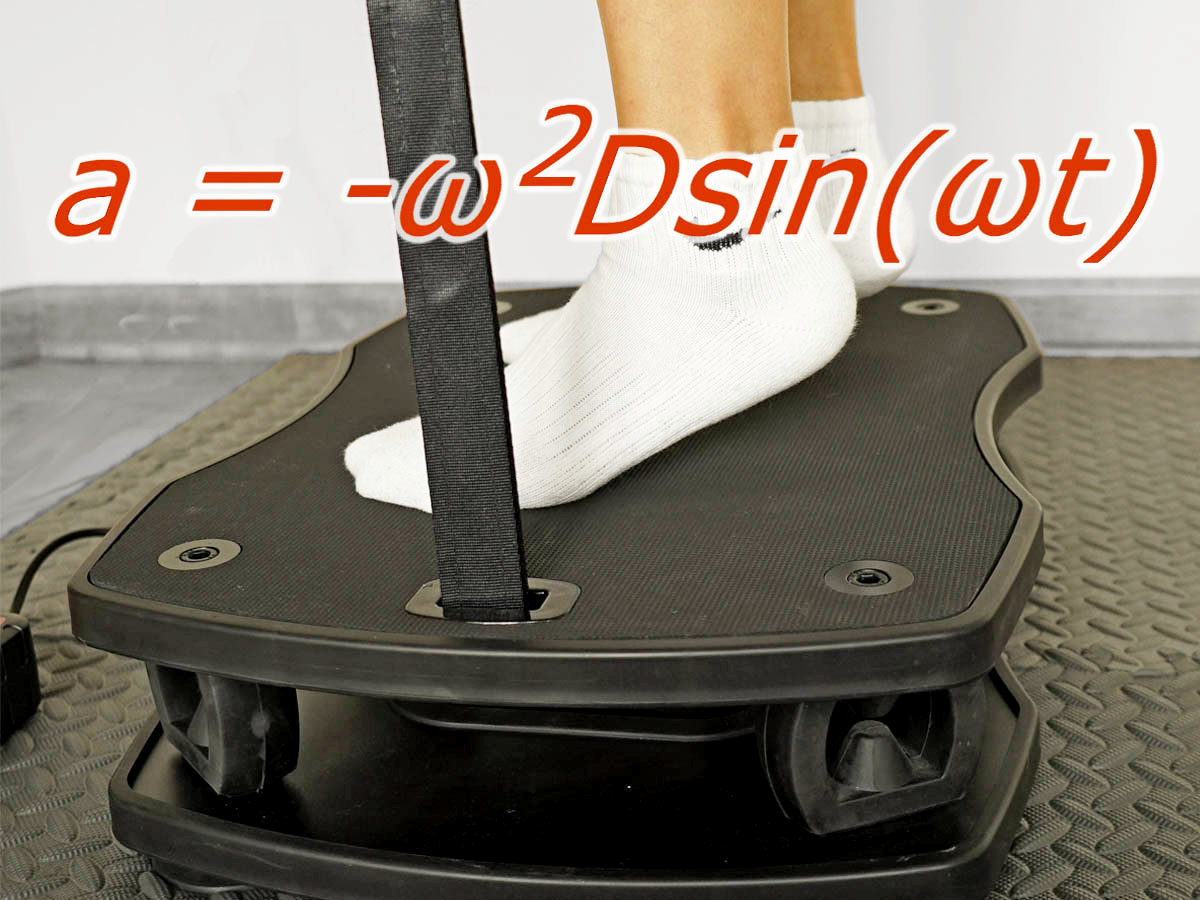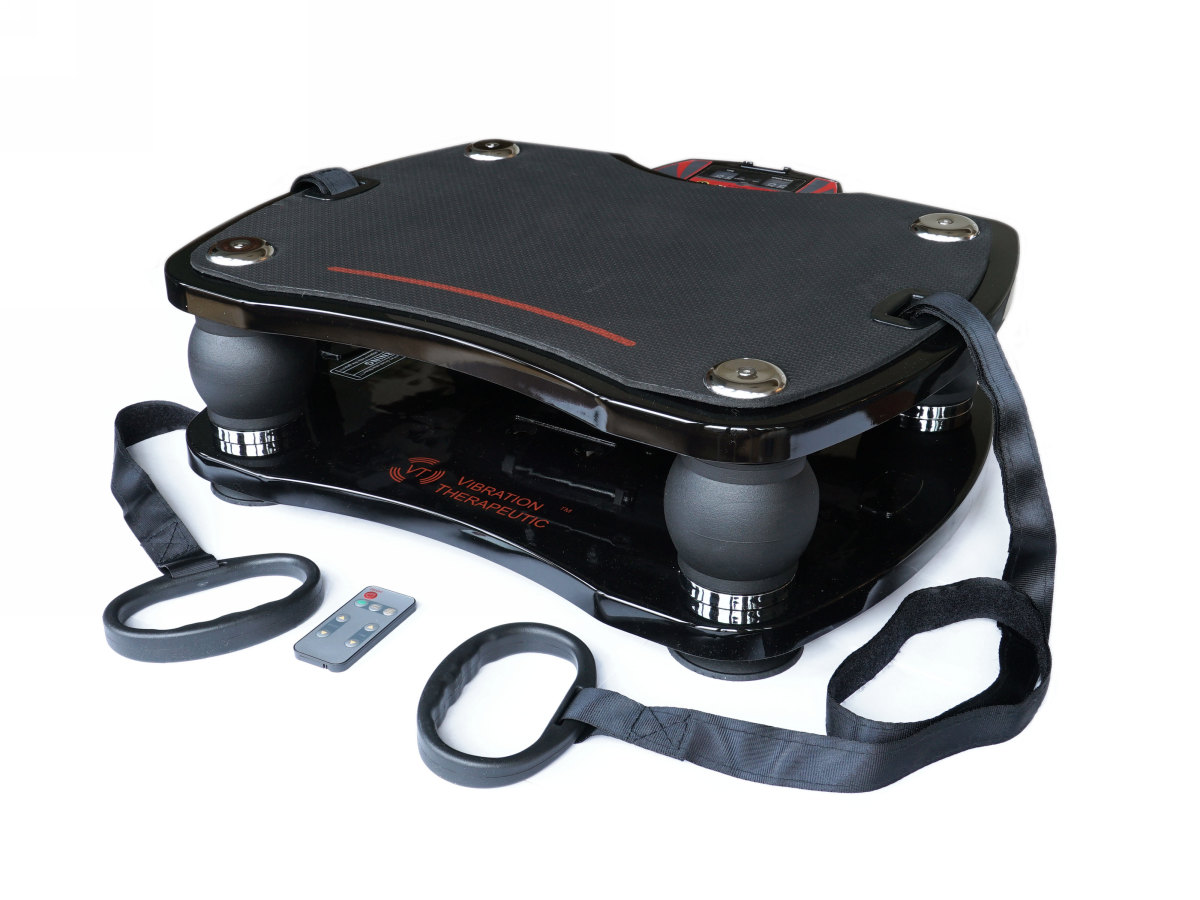Customer Support
devoted to making a better vibration plate
Contact Vibration TherapeuticGold Coast - Jphoto

# Understanding G-force in VibrationSo called G-force, it is actually not a force. G-force is an acceleration rate expressed in the units of G. G is the "acceleration of gravity", equal to 9.81m/s2 at the sea level. In another words, G-force is the acceleration rate divided by G.

Acceleration rate represents velocity change (δv) in a given period of time (δt), or δv divided by δt.

In vibration motion, acceleration rate is calculated from frequency, amplitude, and the time phase of the moving object. Vibration G-force keeps changing its value between the max positive value and the max negative value in every vibration cycle.

For vibration plate, G-force is used as an important vibration intensity indicator. The higher the G-force, the higher the impact the vibration plate can have on the human body.

The simple harmonic vibration model is usually used in physics to analyze and calculate velocity and acceleration rate.

## Acceleration in Simple Harmonic Vibration

We derive our acceleration calculation from a simple harmonic motion equation:

Velocity v = ωDcos(ωt)

Acceleration a = -ω2Dsin(ωt)

D is the displacement from the equilibrium point, ω=2πf is the angular frequency, and t is the time.

Therefore, a=-(2πf)2Dsin(2πft)

In vibration motion, the object moves in alternate directions. The object velocity reaches the maximum value at the equilibrium points and decreases to zero at the peak points. In the meantime, the acceleration changes from zero at the equilibrium points and to the max value at the peak points, alternating between the negative value and the positive value in every cycle. Such a dynamics is expressed by the factor sin(2πft) in the equation.

To understand the dynamics, we need to examine the four time phases of a vibration cycle.

 Phase 1 The object travels from equilibrium point to the highest point (peak). Phase 2 The object change the movement direction at the peak and travel back to the equilibrium point. Phase 3 the object continues to travel to the lowest point (the other peak) Phase 4 The object changes direction again and travel back to the original equilibrium point. This finishes a vibration cycle.

The velocity and acceleration of the object at different points / time phases.

 Object Point Velocity Acceleration At equilibrium Max 0 Phase 1 travel Decrease Negative At up-peak 0 Max Phase 2 travel Increase Positive At equilibrium Max 0 Phase 3 travel Decrease Negative At low-peak 0 Max Phase 4 travel Increase Positive

As the object moves towards the peak point, the acceleration approaches the max negative value. Immediately after the object passes the peak point, the acceleration achieves the max positive value.

The total time of single circle is 1/f. The time of a single phase is ¼ of 1/f. When the object is at the peak point, D = A (Amplitude).

Therefore, at the peak point, the object achieve the maximum positive and negative acceleration rate. The dynamic factor in the equation sin(2πft)=sin(π/2) =1. Therefore,

apeak = ±(2πf)2A

When the object moves close to a peak point, apeak = -(2πf)2A; when the object starts to move away from a peak point, apeak = (2πf)2A

## Peak Acceleration Rate & Phase Acceleration Rate

The peak acceleration rate happens only at the moment the object arrives at the peak point. It is not appropriate to use the peak acceleration rate to evaluate the impact of vibration acceleration to human body. We introduce two acceleration concepts for the evaluation of the vibration acceleration:

1) Peak Acceleration Rate

Peak Acceleration Rate is the max acceleration rate of the object in a phase.

apeak = ±(2πf)2A

2) Phase Acceleration Rate

Phase Acceleration Rate is defined as the AVERAGE between zero and the Peak Acceleration Rate of the phase. For whole body vibration machine, we consider Phase Acceleration Rate better represents vibration acceleration than Peak Acceleration Rate.

Phase 1 & 3, aphase = -2π2f2A

Phase 2 & 4, aphase = 2π2f2A

It is IMPORTANT to note that, for vibration machines, the Amplitude quoted by most manufacturers is actually the Peak-to-Peak Amplitude. The Amplitude in the formula above is the Amplitude as defined in physics, which is half of the Peak-to-Peak Amplitude.

## Gravity Considered in Vertical Vibration Movement

For most whole body vibration machines, the major movement is on vertical direction. Gravity need to be added in the calculation of acceleration. Therefore, the acceleration values for each phase are:

Phase 1, aphase = -2π2f2A + G

Phase 2, aphase = 2π2f2A + G

Phase 3, aphase = -2π2f2A + G

Phase 4, aphase = 2π2f2A + G

These formula calculate the acceleration of the vibration PLATFORM of the machine. However, in vibration exercise, the user's body is not affixed on the vibration platform. When the vibration platform accelerates or decelerates faster than the acceleration of gravity, user's body may not fully stick on the platform. The body can be in projectile motion or free-fall motion.

In phase 1, the platform moves up in decelerated speed, at the point the absolute acceleration rate is greater than G, user's body is in the projectile motion, and the accelerating rate is -G. Although User's body and platform may not arrive at their respective peak positions in the same time, we know in phase 1, the body's acceleration is between -G and 0.

In phase 2, the platform moves down in accelerating speed, at the point the acceleration rate is greater than G, user's body is in free-fall motion, the acceleration rate is G. Although User's body and platform may not fall from their respective peak positions in the same time, we know in phase 2, the body acceleration is between 0 and G.

In phase 3, the platform continue moves down in decelerating speed, user's body continues with free fall and then catch up with the platform at a certain point. The body acceleration rate is between G and -2π2f2A + G.

In phase 4, the platform moves up in accelerating speed, user's body stays on the platform in the entire phase. The body acceleration rate is 2π2f2A + G

## Final G-Force Formula

Our goal is to evaluate the impact of the highest Phase Acceleration Rate on user's body, which is Phase 4 Acceleration Rate.

Phase 4 Acceleration Rate = 2π2f2A + G

Phase 4 Acceleration Rate converted to G-force (divided by G):

Phase 4 G-force =2π2f2A/G + 1

Also in Phase 4, for reference, the maximum G-force can be calculated as

Phase 4 Peak G-force = (2πf)2A/G +1

Phase 4 G-force is the acceleration values we use to evaluate acceleration of the vertical movement of whole body vibration machine.

## Impact of Vibration G-force on Human Body

In vibration exercise, vibration acceleration creates additional force on human body. This additional force is called acceleration force. It is calculated as the body weight multiplied by the G-force.

For example, a 200LB person stands on a running vibration plate that has the maximum G-force of 3. In the 4th phase of a vibration circle when G-force reaches the maximum value, the person will feel the weight increased to 2x200LB=600LB, the peak acceleration force. However, the person will not perceive such a heavy weight, because it only lasts a tiny fraction of a second time.

## G-Force Calculation Examples

#### G-Force of Model VT003FFor Model VT003F vibration plate , the max G-force occurs at the frequency 40Hz, and peak-to-peak amplitude 1.32mm (A=0.66mm), the acceleration rate are calculate as

Phase 4 G-force =2x3.142x402x0.00066/9.8+1=3.13

#### G-Force of Model VT007For Model VT007 vibration plate , the max G-force occurs at the frequency 40Hz, and peak-to-peak amplitude 1.78mm (A=0.89mm), the acceleration rate are calculate as

Phase 4 G-force =2x3.142x402x0.00089/9.8+1=3.86

#### G-force of Model VT027For Model VT027 vibration plate , given the highest frequency 14Hz, and the highest peak-to-peak amplitude is 10mm (A=5mm), the acceleration rates are calculate as

Phase 4 G-force =2x3.142x142x0.005/9.8+1=2.97

#### G-force of Model VT020-3For Model VT020-3 vibration plate , given the highest frequency 15Hz, and the highest (peak-to-peak) amplitude 10mm (0.003m), the acceleration rates are calculate as

Phase 4 G-force = 2x3.142x152x0.005/9.8+1=3.26